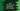# java.util.Arrays.fill method explanation with examples## java.util.Arrays.fill method explanation with examples:

Arrays class of java.util package provides different useful array related methods. In this post, I will show you how to use the fill() method of Arrays class with examples.

This method is useful if you want to fill an array with some specific value. It is available for all different data types. You can also fill an array in a range with this method.

Let’s check how it is defined.

### Definition of Arrays.fill method:

The Arrays.fill method is defined as like below:

``fill(T[] arr, T v)``

Here, T is the data type, arr is the array to use and v is the value to fill in the array.

We can also pass the from and to index to this method:

``fill(T[] arr, int from, int to, T v)``

Here, from is the index of the first element(inclusive) to start the filling. And, to is the index of the last element(exclusive) to stop the filling.

### Exceptions:

It can throw any of these exceptions:

#### IllegalArgumentException:

If from is greater than to

#### ArrayIndexOutOfBoundsException:

If from is less than 0 or to > array length

#### ArrayStoreException:

If the specified element can’t be stored in the array.

#### Boolean:

These methods are used with a boolean array and used to fill a boolean value in the array:

``````fill(boolean[] arr, boolean v)
fill(boolean[] arr, int from, int to, boolean v)``````

#### byte:

These methods are used with a byte array and used to fill a byte value in the array:

``````fill(byte[] arr, byte v)
fill(byte[] arr, int from, int to, byte v)``````

#### char:

These methods are used with a char array and used to fill a char value in the array:

``````fill(char[] arr, char v)
fill(char[] arr, int from, int to, char v)``````

#### double:

These methods are used with a double array and used to fill a double value in the array:

``````fill(double[] arr, char v)
fill(double[] arr, int from, int to, double v)``````

#### float:

These methods are used with a float array and used to fill a float value in the array:

``````fill(float[] arr, float v)
fill(float[] arr, int from, int to, float v)``````

#### int:

These methods are used with a int array and used to fill a int value in the array:

``````fill(int[] arr, int v)
fill(int[] arr, int from, int to, int v)``````

#### long:

These methods are used with a long array and used to fill a long value in the array:

``````fill(long[] arr, long v)
fill(long[] arr, int from, int to, long v)``````

#### object:

These methods are used with a object array and used to fill a object value in the array:

``````fill(object[] arr, object v)
fill(object[] arr, int from, int to, object v)``````

#### short:

These methods are used with a short array and used to fill a short value in the array:

``````fill(short[] arr, short v)
fill(short[] arr, int from, int to, short v)``````

### Example of Arrays.fill:

Let me show you an example of Arrays.fill method with an integer array:

``````import java.util.Arrays;

class Main {

public static void main(String[] args) {
int[] arr = new int;

Arrays.fill(arr, -1);

System.out.println(Arrays.toString(arr));
}
}``````

In this example, we created an array of integers arr with size 10. We are using Arrays.fill method to fill the array with -1. The last line is using toString method of Arrays class to convert the array to a string.

It will give the following output:

``[-1, -1, -1, -1, -1, -1, -1, -1, -1, -1]``

As you can see here, it inserted -1 to all elements of the array.

### Example of Arrays.fill with a range:

Let’s use from and to index with Arrays.fill method:

``````import java.util.Arrays;

class Main {

public static void main(String[] args) {
int[] arr = new int;

Arrays.fill(arr, 1, 3, -1);

System.out.println(Arrays.toString(arr));
}
}``````

In this example, we are passing 1 as the from index and 3 as the to index to Arrays.fill. So, it will fill -1 from index 1 to index 2.

It will print:

``[0, -1, -1, 0, 0, 0, 0, 0, 0, 0]``

### Example of Arrays.fill with objects:

Let’s try Arrays.fill method with an array of objects:

``````import java.util.Arrays;

class Student {
String name;
int age;

Student(String n, int a) {
this.name = n;
this.age = a;
}

@Override
public String toString() {
return "Student{" +
"name='" + name + '\'' +
", age=" + age +
'}';
}
}

class Main {

public static void main(String[] args) {
Student emptyStudent = new Student("", -1);

Student[] studentsArray = new Student;

Arrays.fill(studentsArray, emptyStudent);

System.out.println(Arrays.toString(studentsArray));
}
}``````

Here,

• Student is a class to hold the info of a Student. It can hold a string name and an integer age.
• emptyStudent is an object of Student with an empty string as the name and -1 as the age.
• We are creating an array of Student objects of size 5.
• The Arrays.fill method is used to fill the array with emptyStudent object.

If you run this program, it will print:

``[Student{name='', age=-1}, Student{name='', age=-1}, Student{name='', age=-1}, Student{name='', age=-1}, Student{name='', age=-1}]``

As you can see here, it is filled with the object emptyStudent.

### Example of Arrays.fill with objects in a range:

Let’s modify the above example to fill the array in a range:

``````import java.util.Arrays;

class Student {
String name;
int age;

Student(String n, int a) {
this.name = n;
this.age = a;
}

@Override
public String toString() {
return "Student{" +
"name='" + name + '\'' +
", age=" + age +
'}';
}
}

class Main {

public static void main(String[] args) {
Student emptyStudent = new Student("", -1);

Student[] studentsArray = new Student;

Arrays.fill(studentsArray, 0, 3, emptyStudent);

System.out.println(Arrays.toString(studentsArray));
}
}``````

In this program, we are using Arrays.fill to fill between index 0 to 3. It will print:

``[Student{name='', age=-1}, Student{name='', age=-1}, Student{name='', age=-1}, null, null]``

It filled emptyStudent for the indices 0, 1 and 2 of the studentsArray.